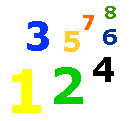# Estimating Differences

Estimating Differences Lesson & Worksheet - My Schoolhouse - Online Learning

You can estimate to find out "about how many" when subtracting as well as when adding.  This is also called rounding.

You can estimate the differences of two-digit and three-digit numbers.

Look at the examples below.

 77 Rounds to 80 31 Rounds to -  30 50

The estimated difference of 77 - 31 is 50.

 459 Rounds to 500 287 Rounds to -  300 200

The estimated difference of 495 - 287 is 200.

Practice estimating the difference with two-digit and three-digit numbers.

 67 Rounds to 52 Rounds to -  32 Rounds to - -  26 Rounds to -467 Rounds to 345 Rounds to -  159 Rounds to - -  189 Rounds to -81 Rounds to 47 Rounds to -  32 Rounds to - -  16 Rounds to -269 Rounds to 666 Rounds to -  115 Rounds to - -  421 Rounds to -27 Rounds to 94 Rounds to -  11 Rounds to - -  37 Rounds to -525 Rounds to 813 Rounds to -  210 Rounds to - -  199 Rounds to -38 Rounds to 73 Rounds to -  26 Rounds to - -  19 Rounds to -